# Recent Activity

## Kriesell's Conjecture ★★

Author(s): Kriesell

Conjecture   Letbe a graph and let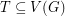such that for any pair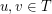there are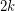edge-disjoint paths fromtoin. Thencontainsedge-disjoint trees, each of which contains.

## 2-colouring a graph without a monochromatic maximum clique ★★

Author(s): Hoang; McDiarmid

Conjecture   Ifis a non-empty graph containing no induced odd cycle of length at least, then there is a-vertex colouring ofin which no maximum clique is monochromatic.

Keywords: maximum clique; Partitioning

## Almost all non-Hamiltonian 3-regular graphs are 1-connected ★★

Author(s): Haythorpe

Conjecture   Denote bythe number of non-Hamiltonian 3-regular graphs of size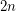, and similarly denote by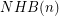the number of non-Hamiltonian 3-regular 1-connected graphs of size.

Is it true that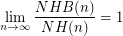?

## Erdős–Faber–Lovász conjecture ★★★

Author(s): Erdos; Faber; Lovasz

Conjecture   Ifis a simple graph which is the union ofpairwise edge-disjoint complete graphs, each of which hasvertices, then the chromatic number ofis.

Keywords: chromatic number

## Are there only finite Fermat Primes? ★★★

Author(s):

Conjecture   A Fermat prime is a Fermat numberthat is prime. The only known Fermat primes are F_0 =3,F_1=5,F_2=17,F_3 =257 ,F_4=65537 It is unknown if other fermat primes exist.

Keywords:

## Are all Fermat Numbers square-free? ★★★

Author(s):

Conjecture   Are all Fermat NumbersSquare-Free?

Keywords:

## Choosability of Graph Powers ★★

Author(s): Noel

Question  (Noel, 2013)   Does there exist a function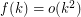such that for every graph,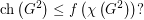## Erdős-Posa property for long directed cycles ★★

Author(s): Havet; Maia

Conjecture   Letbe an integer. For every integer, there exists an integersuch that for every digraph, eitherhas apairwise-disjoint directed cycles of length at least, or there exists a setof at most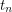vertices such that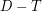has no directed cycles of length at least.

Keywords:

## Large acyclic induced subdigraph in a planar oriented graph. ★★

Author(s): Harutyunyan

Conjecture   Every planar oriented graphhas an acyclic induced subdigraph of order at least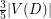.

Keywords:

## Polignac's Conjecture ★★★

Author(s): de Polignac

Conjecture   Polignac's Conjecture: For any positive even number n, there are infinitely many prime gaps of size n. In other words: There are infinitely many cases of two consecutive prime numbers with difference n.

In particular, this implies:

Conjecture   Twin Prime Conjecture: There are an infinite number of twin primes.

Keywords: prime; prime gap

## Alexa's Conjecture on Primality ★★

Author(s): Alexa

Definition   Letbe the unique integer (with respect to a fixed) such that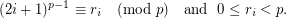Conjecture   A natural number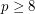is a prime iffKeywords: primality

## P vs. BPP ★★★

Author(s): Folklore

Conjecture   Can all problems that can be computed by a probabilistic Turing machine (with error probability < 1/3) in polynomial time be solved by a deterministic Turing machine in polynomial time? That is, does P = BPP?

Keywords: BPP; circuit complexity; pseudorandom generators

## Goldbach conjecture ★★★★

Author(s): Goldbach

Conjecture   Every even integer greater than 2 is the sum of two primes.

## Goldberg's conjecture ★★★

Author(s): Goldberg

The overfull parameter is defined as follows:Conjecture   Every graphsatisfies.

Keywords: edge-coloring; multigraph

## Cyclic spanning subdigraph with small cyclomatic number ★★

Author(s): Bondy

Conjecture   Letbe a digraph all of whose strong components are nontrivial. Thencontains a cyclic spanning subdigraph with cyclomatic number at most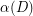.

Keywords:

## inverse of an integer matrix ★★

Author(s): Gregory

Question   I've been working on this for a long time and I'm getting nowhere. Could you help me or at least tell me where to look for help. Suppose D is an m-by-m diagonal matrix with integer elements all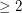. Suppose X is an m-by-n integer matrix. Consider the partitioned matrix M = [D X]. Obviously M has full row rank so it has a right inverse of rational numbers. The question is, under what conditions does it have an integer right inverse? My guess, which I can't prove, is that the integers in each row need to be relatively prime.

## Minimum number of arc-disjoint transitive subtournaments of order 3 in a tournament ★★

Author(s): Yuster

Conjecture   Ifis a tournament of order, then it containsarc-disjoint transitive subtournaments of order 3.

Keywords:

## Arc-disjoint directed cycles in regular directed graphs ★★

Author(s): Alon; McDiarmid; Molloy

Conjecture   Ifis a-regular directed graph with no parallel arcs, thencontains a collection ofarc-disjoint directed cycles.

Keywords:

## Jacob Palis Conjecture(Finitude of Attractors)(Dynamical Systems) ★★★★

Author(s):

Conjecture   Let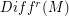be the space ofDiffeomorphisms on the connected , compact and boundaryles manifold M and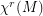the space ofvector fields. There is a dense set() such that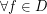exhibit a finite number of attractor whose basins cover Lebesgue almost all ambient space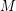This is a very Deep and Hard problem in Dynamical Systems . It present the dream of the dynamicist mathematicians .

Keywords: Attractors , basins, Finite

## Closing Lemma for Diffeomorphism (Dynamical Systems) ★★★★

Author(s): Charles Pugh

Conjecture   Letand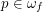. Then for any neighborhood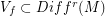there issuch thatis periodic point of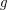There is an analogous conjecture for flows (vector fields . In the case of diffeos this was proved by Charles Pugh for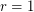. In the case of Flows this has been solved by Sushei Hayahshy for. But in the two cases the problem is wide open for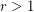Keywords: Dynamics , Pertubation